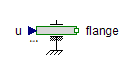Multiplex Real - MapleSim Help

Multiplex Real

Multiplexer for Real SignalsDescription The Real multiplexer (or Multiplex Real) component combines Real input vectors into a Real output vector. The $n$ parameter specifies the dimension of each input vector. It is a vector of positive integers. The sum of the dimensions is the dimension of the output port. For example, if $n=\left[2,1,4\right]$, there will be 3 inputs ports with dimensions 2, 1, and 4, respectively. The output port has dimension 7. Input port 1 is routed to output signals 1 to 2, input port 2 is routed to output signal 3, and input port 3 is routed to output signals 4 to 7.

Connections

 Name Description Modelica ID $\mathrm{ui_j}$ Real input vectors ui_j $y$ Real output vector y

Parameters

 Name Default Units Description Modelica ID $n$ $\left[1,1,1\right]$ Array of dimensions of input connectors n

 Modelica Standard Library The component described in this topic is from the Modelica Standard Library. To view the original documentation, which includes author and copyright information, click here.